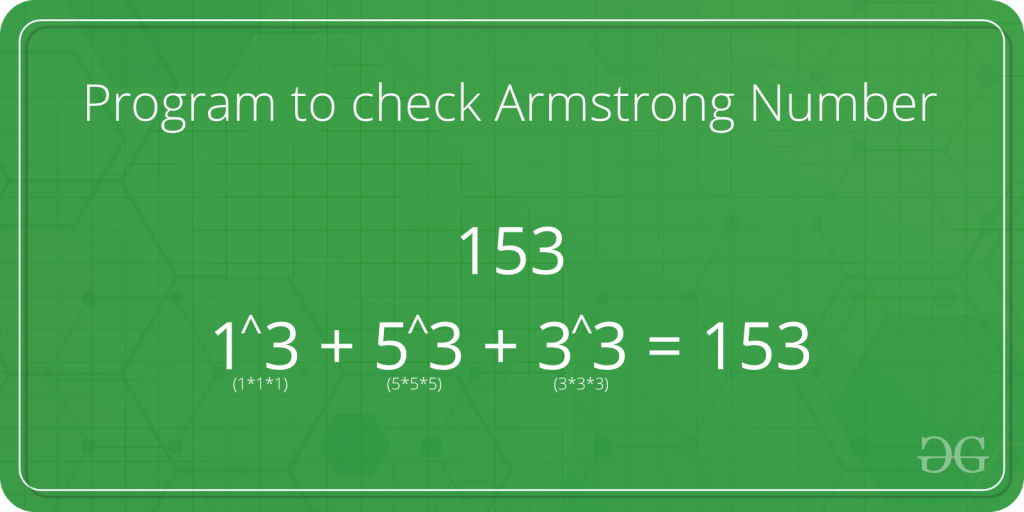# C Program to check Armstrong Number

Given a number x, determine whether the given number is Armstrong number or not.A positive integer of n digits is called an Armstrong number of order n (order is number of digits) if.

```abcd... = pow(a,n) + pow(b,n) + pow(c,n) + pow(d,n) + ....
```

Example:

```Input : 153
Output : Yes
153 is an Armstrong number.
1*1*1 + 5*5*5 + 3*3*3 = 153

Input : 120
Output : No
120 is not a Armstrong number.
1*1*1 + 2*2*2 + 0*0*0 = 9

Input : 1253
Output : No
1253 is not a Armstrong Number
1*1*1*1 + 2*2*2*2 + 5*5*5*5 + 3*3*3*3 = 723

Input : 1634
Output : Yes
1*1*1*1 + 6*6*6*6 + 3*3*3*3 + 4*4*4*4 = 1634
```

## C++

 `// C++ program to determine whether the number is ` `// Armstrong number or not ` `#include ` `using` `namespace` `std; ` ` `  `/* Function to calculate x raised to the power y */` `int` `power(``int` `x, unsigned ``int` `y) ` `{ ` `    ``if``( y == 0) ` `        ``return` `1; ` `    ``if` `(y%2 == 0) ` `        ``return` `power(x, y/2)*power(x, y/2); ` `    ``return` `x*power(x, y/2)*power(x, y/2); ` `} ` ` `  `/* Function to calculate order of the number */` `int` `order(``int` `x) ` `{ ` `    ``int` `n = 0; ` `    ``while` `(x) ` `    ``{ ` `        ``n++; ` `        ``x = x/10; ` `    ``} ` `    ``return` `n; ` `} ` ` `  `// Function to check whether the given number is ` `// Armstrong number or not ` `bool` `isArmstrong(``int` `x) ` `{ ` `    ``// Calling order function ` `    ``int` `n = order(x); ` `    ``int` `temp = x, sum = 0; ` `    ``while` `(temp) ` `    ``{ ` `        ``int` `r = temp%10; ` `        ``sum += power(r, n); ` `        ``temp = temp/10; ` `    ``} ` ` `  `    ``// If satisfies Armstrong condition ` `    ``return` `(sum == x); ` `} ` ` `  `// Driver Program ` `int` `main() ` `{ ` `    ``int` `x = 153; ` `    ``cout << isArmstrong(x) << endl; ` `    ``x = 1253; ` `    ``cout << isArmstrong(x) << endl; ` `    ``return` `0; ` `} `

Please refer complete article on Program for Armstrong Numbers for more details!

My Personal Notes arrow_drop_up
Article Tags :

Be the First to upvote.

Please write to us at contribute@geeksforgeeks.org to report any issue with the above content.